## Character Counting in Excel: Cell & Range (Easy Guide)

In the vast world of Excel, understanding the intricacies of data manipulation is vital. One such aspect is counting characters, specific characters, or certain text within cells or a range of cells. This guide will enlighten you on the step-by-step ways to achieve this. Whether you're a beginner or an Excel wizard, there's always something new to learn!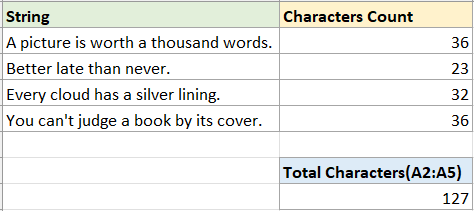### Characters Counts

First, let's start with the most common case: counting the number of characters in a single cell or a range of cells.

#### Count characters in a single cell

To count characters in a single cell, you can use the LEN function—which counts letters, numbers, characters and all spaces in a cell.

##### Step 1: Select a blank cell and use the LEN function

In this case, I want to count the characters in cell A2, please use LEN function as below, then press Enter key.

``=LEN(A2)``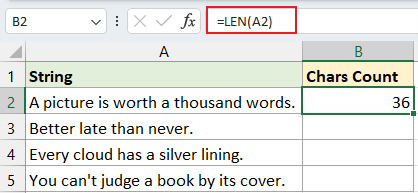##### Step 2 (Optional): Drag auto fill handle over the cells you want to count characters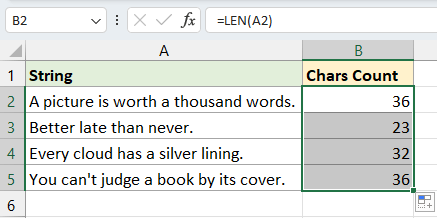For swift and precise character frequency tracking within a string, turn to Kutools for Excel's COUNTCHAR function. Beyond this feature, Kutools offers dozens of functions that simplify complex calculations with ease. Experience the unmatched efficiency of Kutools by downloading it today!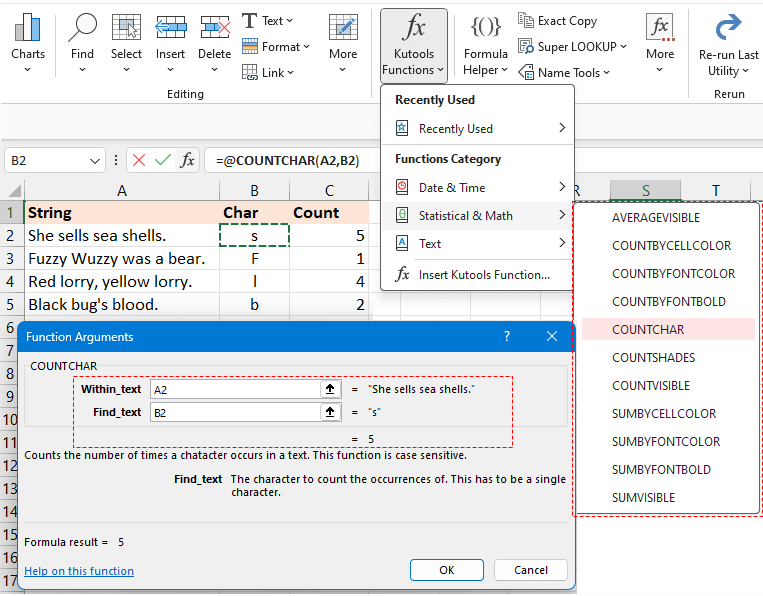#### Count characters in a range of cells

To aggregate the count across multiple cells, you can use the SUMPRODUCT and LEN functions together.

For example, to count the total characters in range A2:A5, use the below formula, then press Enter key to get the count:

``=SUMPRODUCT(LEN(A2:A5))``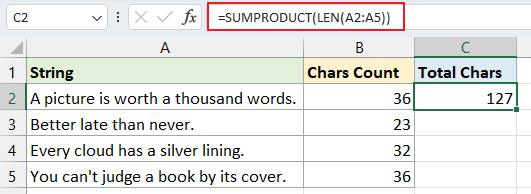Notes:
• You can use the below formula to tally the total characters in range A2:A5.
`` =SUM(LEN(A2:A5))``
However, if in versions prior to Excel 2019, ensure you press Shift + Ctrl + Enter simultaneously to obtain the accurate outcome.
• If you need to count the total characters in several discontinuous cells, such as cells A2 and A5, the above formulas won't work correctly. Please use the following formula instead:
``=SUM(LEN(A2),LEN(A5))``

### Certain, specific characters counts

In some case, you may want to count one certain character in a string or a range of cells. However, distinguishing between case-sensitive and case-insensitive counting can be crucial depending on your data needs. This section will introduce the methods on solving these problems.

#### Count specific characters with case sensitivity in cell or a range

For counting specific character with case sensitivity, here we provides two different methods.

Method 1: Using formula combined LEN and SUBSTITUTE functions

Method 2: Using Kutools for Excel with clicks

##### Method 1: Using formula combined LEN and SUBSTITUTE functions
• Count specific character with case sensitivity in a cell

For example, to count the number of character "s" in cell A2, please use the below formula and press Enter key:

``=LEN(A2)-LEN(SUBSTITUTE(A2,"s",""))``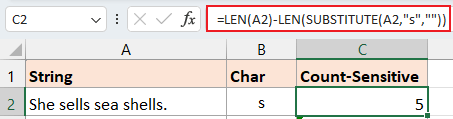Formula explanation:
• LEN(A2): Count the total characters in cell A2.
• SUBSTITUTE(A2,"s",""): Replace all character "s" with empty.
• LEN(SUBSTITUTE(A2,"s","")):Get the length of characters in A2 without character "s".
• LEN(A2)-LEN(SUBSTITUTE(A2,"s","")): The total characters in cell A2 subtract the length of characters in A2 without the character "s". The result will be the number of character "s" in A2.
Note: You can use a cell reference to specify the character in the formula, which will automatically adjust as you fill the formula using the auto-fill handle.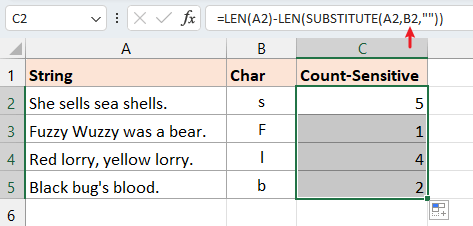• Count specific character with case sensitivity in a range

If you want to count the character "s" in a range A2:A5, please use below formula and press Enter key:

``=SUMPRODUCT(LEN(A2:A5) - LEN(SUBSTITUTE(A2:A5,"s", "")))``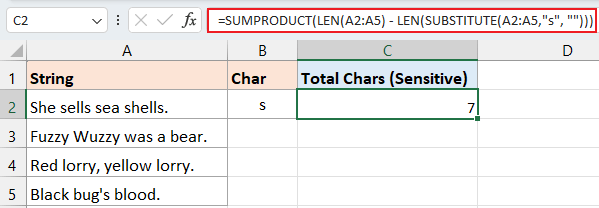##### Method 2: Using Kutools for Excel with clicks

To effortlessly pinpoint the frequency of a specific character within a string, lean on Kutools for Excel's COUNTCHAR function. It's a superior solution, allowing you to instantly ascertain the count of any character within a cell without having to remember complex formulas.

After free installing Kutools for Excel, select a blank cell and click Kutools > Kutools Functions > Statistical & Math > COUNTCHAR. Then in the Function Argument dialog, please:

1. Click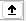in the Within_text textbox to select the cell that you want to count specific character in.
2. Clickin the Find_text textbox to select the cell that reference to the specific character you want to count. (Or type the specific character in the Find_text textbox.) Then click OK.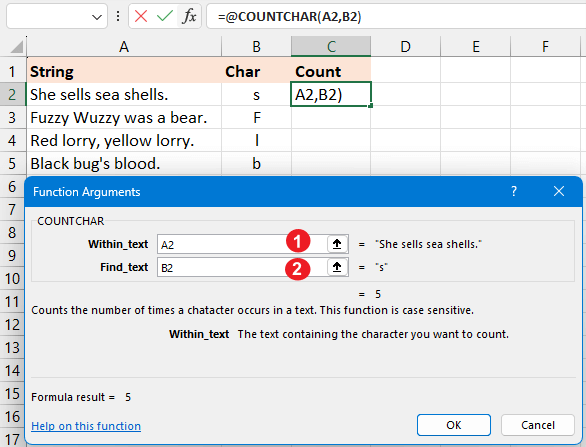If in the Find_text text box, you use the reference cell, you can drag the fill handle over other cells to apply this formula and obtain the counts.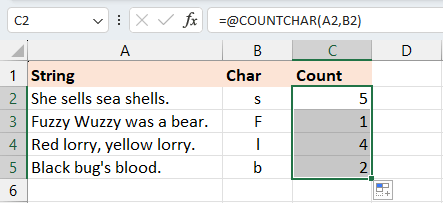If you want to get the total number of character "s" in a range A2:A5, go to the Find_text textbox and type "s", then drag auto fill handle down to count the character "s" in each cell from A2:A5, then using SUM function to get the total.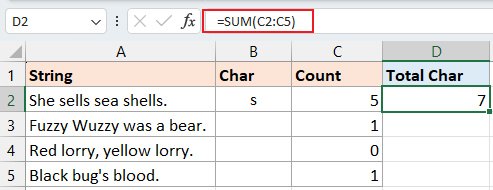Boost your Excel efficiency with an arsenal of functions and formulas tailor-made for intricate tasks. Whether you need to count specific text, tally colors, or identify unique values, Kutools for Excel has got you covered. Experience this productivity-enhancing toolkit for yourself—download Kutools for Excel today!

#### Count specific characters with case insensitivity in a cell or a range

• Count specific character with case insensitivity in a cell

If you want to count the characters "s" or "S" in cell A2, you can use the following formula and press Enter key:

``=LEN(A2) - LEN(SUBSTITUTE(UPPER(A2), UPPER("s"),""))``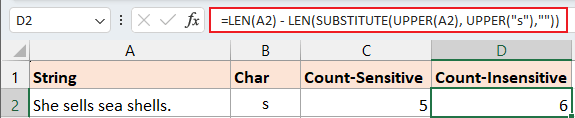Formula explanation:
• LEN(A2): Count the total characters in cell A2.
• UPPER("s"): Change "s" to "S".
• UPPER(A2): Change all letters in the cell A2 to upper case.
• SUBSTITUTE(UPPER(A2), UPPER("s"),""): Replace all character "S" with empty.
• LEN(SUBSTITUTE(UPPER(A2), UPPER("s"),"")):Get the length of characters in A2 without character "s" and "S".
• LEN(A2)-LEN(SUBSTITUTE(A2,"s","")): The total characters in cell A2 subtract the length of characters in A2 without the character "s" and "S". The result will be the number of character "s" and "S" in A2.
Note: You can use a cell reference to specify the character in the formula, which will automatically adjust as you fill the formula using the auto-fill handle.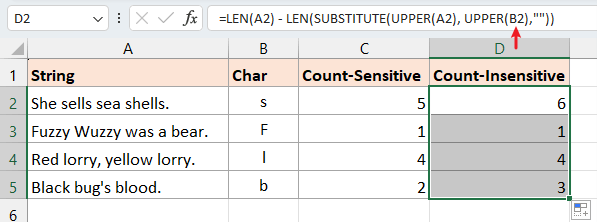• Count specific character with case insensitivity in a range

If you want to count the total number of character "s" and "S" in a range A2:A5, please use below formula and press Enter key:

``=SUMPRODUCT(LEN(A2:A5) - LEN(SUBSTITUTE(UPPER(A2:A5), UPPER("s"), "")))``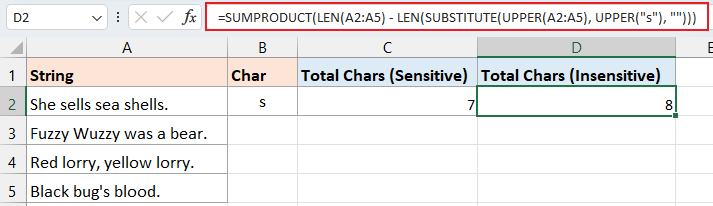### Count certain text

At times, beyond just tallying a particular character, you might find the need to quantify specific text within a cell or across a range. In such scenarios, being mindful of case sensitivity is crucial as it can yield varying outcomes.

#### Count certain text with case sensitivity in a cell or a range

For counting a certain text with case sensitivity, here we also provides two different methods.

Method 1: Using formula combined LEN and SUBSTITUTE functions

Method 2: Using Kutools for Excel with clicks

##### Method 1: Using formula combined LEN and SUBSTITUTE functions
• Count certain word with case sensitivity in a cell

For example, to count the number of the word "see" in cell A2, please use the below formula and press Enter key:

``=(LEN(A2) - LEN(SUBSTITUTE(A2, "see", ""))) / LEN("see")``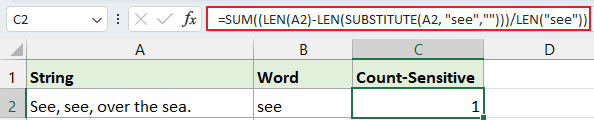Note: You can use a cell reference to specify the certain text in the formula, which will automatically adjust as you fill the formula using the auto-fill handle.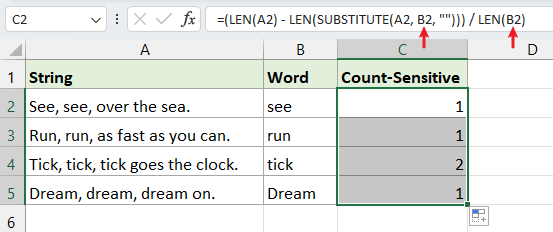• Count certain word with case sensitivity in a range

If you want to count the word "and" in a range A2:A5, please use below formula and press Enter key:

``=SUMPRODUCT((LEN(A2:A5) - LEN(SUBSTITUTE(A2:A5, "and", ""))) / LEN("and"))``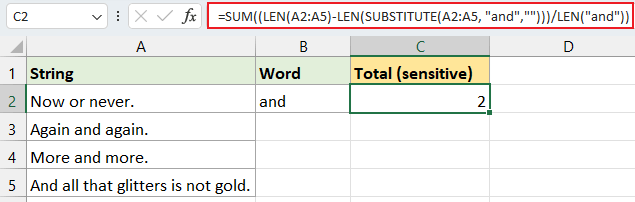##### Method 2: Using Kutools for Excel with clicks

Instead of grappling with lengthy and intricate formulas, there's a more straightforward method to swiftly determine the count of a specific word in a cell or range in Excel. Look no further than Kutools for Excel's Count the number of a Word tool. Just a few clicks, and you're set to go. Simplify your tasks with this efficient tool and sidestep the complexities of traditional formulas.

After installing Kutools for Excel, select a blank cell and click Kutools > Formula Helper> Statistical > Count the number of a word. Then in the Function Argument dialog, please:

• Click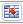in the Text textbox to select the cell that you want to count specific word in.
• Clickin the Word textbox to select the cell that reference to the specific word you want to count. (Or type the specific word in the Word textbox.) Then click OK.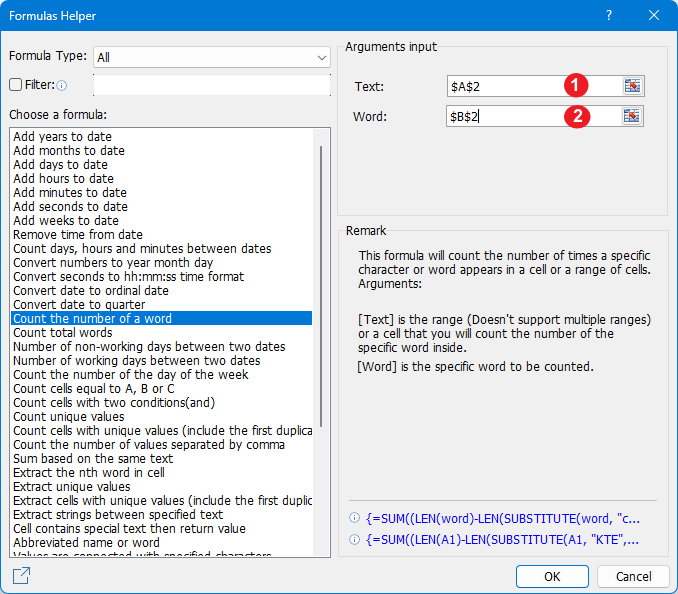If in the Text textbox, you use the reference cell you can drag the fill handle over other cells to apply this formula and obtain the counts.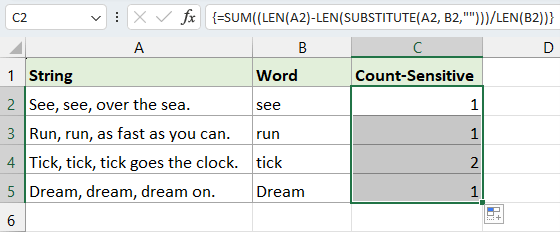If you want to get the total number of the word "and" in a range A2:A5, just select the range A2:A5 in the Text textbox in the Formula Helper.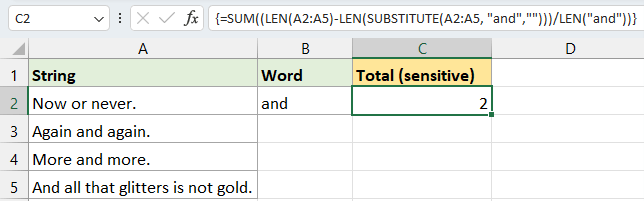Elevate your Excel game with Kutools, a powerhouse of functions and formulas designed for nuanced tasks. From counting characters to tallying colors and tracking unique values, Kutools for Excel stands ready to streamline your workflow. Don't just take our word for it - download and witness the Kutools difference firsthand!

#### Count certain text with case insensitivity in cell or a range

• Count certain text with case insensitivity in a cell

To tally the occurrences of the word "see" in cell A2 without considering case distinctions (whether it's "SEE", "see", "SeE", etc.), you can employ the formula below. After entering it, simply press Enter key:

``=(LEN(A2)-LEN(SUBSTITUTE(UPPER(A2), UPPER("see"),""))) / LEN("see")``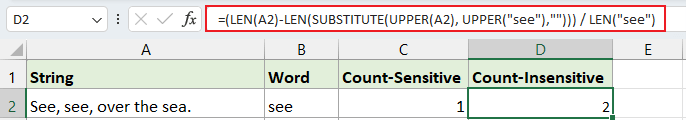Note: You can use a cell reference to specify the word in the formula, which will automatically adjust as you fill the formula using the auto-fill handle.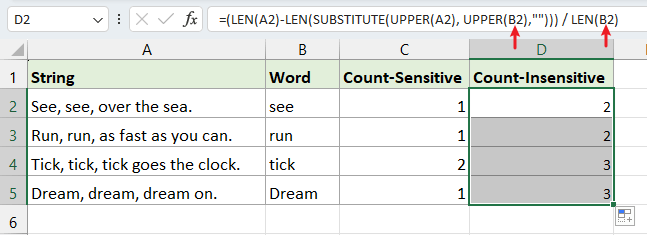• Count specific character with case insensitivity in a range

If you want to count the total number of word "and" with case-insensitive in a range A2:A5, please use below formula and press Enter key:

``=SUMPRODUCT((LEN(A2:A5) - LEN(SUBSTITUTE(UPPER(A2:A5), UPPER("and"), ""))) / LEN(UPPER("and")))``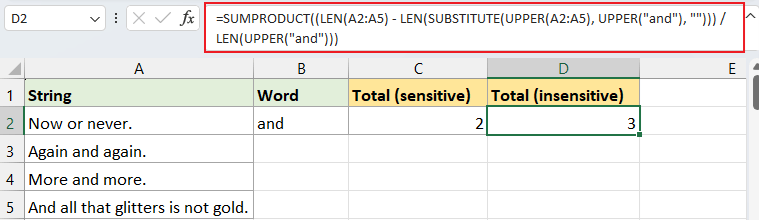The insights shared above outline methods to count characters, specific characters, and particular text in Excel. I trust this information serves you well. For more game-changing Excel strategies that can elevate your data management, explore further here..

### The Best Office Productivity Tools

#### Kutools for Excel - Helps You To Stand Out From Crowd

 Popular Features: Find, Highlight or Identify Duplicates  |  Delete Blank Rows  |  Combine Columns or Cells without Losing Data  |  Round without Formula ... Super VLookup: Multiple Criteria  |  Multiple Value  |  Across Multi-Sheets  |  Fuzzy Lookup... Adv. Drop-down List: Easy Drop Down List  |  Dependent Drop Down List  |  Multi-select Drop Down List... Column Manager: Add a Specific Number of Columns  |  Move Columns  |  Toggle Visibility Status of Hidden Columns  |  Compare Columns to Select Same & Different Cells ... Featured Features: Grid Focus  |  Design View  |  Big Formula Bar  |  Workbook & Sheet Manager | Resource Library (Auto Text)  |  Date Picker  |  Combine Worksheets  |  Encrypt/Decrypt Cells  |  Send Emails by List  |  Super Filter  |  Special Filter (filter bold/italic/strikethrough...) ... Top 15 Toolsets:  12 Text Tools (Add Text, Remove Characters ...)  |  50+ Chart Types (Gantt Chart ...)  |  40+ Practical Formulas (Calculate age based on birthday ...)  |  19 Insertion Tools (Insert QR Code, Insert Picture from Path ...)  |  12 Conversion Tools (Numbers to Words, Currency Conversion ...)  |  7 Merge & Split Tools (Advanced Combine Rows, Split Excel Cells ...)  |  ... and more

Kutools for Excel Boasts Over 300 Features, Ensuring That What You Need is Just A Click Away...#### Office Tab - Enable Tabbed Reading and Editing in Microsoft Office (include Excel)

• One second to switch between dozens of open documents!
• Reduce hundreds of mouse clicks for you every day, say goodbye to mouse hand.
• Increases your productivity by 50% when viewing and editing multiple documents.
• Brings Efficient Tabs to Office (include Excel), Just Like Chrome, Edge and Firefox.No ratings yet. Be the first to rate!
This comment was minimized by the moderator on the site
To count all characters in a worksheet: - Rather than bother copying to Word, just use formula
' =SUMPRODUCT(LEN(A1:B5)) '.
It works, try it!!
In fact SUM(LEN(A1:B5)) will probably crash with #VALUE! error if used over large ranges, or a lot of characters are in each cell,
particularly in older versions of Excel like Excel2000.

So if you are getting #VALUE! errors when using ' SUM(LEN(A1:B5)) ', switch to SUMPRODUCT.
I prefer this to tediously copying to Word, because with SUMPRODUCT :-

1) It works over large ranges easily, on cell formating of any kind

2) You can deduct parts of a range that may not be relevant (e.g. a cell, row or column that contains personal notes not relevant to the data you want to count)
e.g. =SUMPRODUCT(LEN(B1:L5799)) - LEN(C4) removes the count of characters in Cell C4 from the whole range B1:L5799
=SUMPRODUCT(LEN(B1:L5799)) - SUMPRODUCT(LEN(B462:L462)) removes the count of characters in Row 462 from the whole range B1:L5799
=SUMPRODUCT(LEN(B1:L5799)) - SUMPRODUCT(LEN(D1:D5799)) removes the count of characters in Col. D from the whole range B1:L5799

3) You can add ranges on more than one sheet, so without pasting every sheet to Word, you can get a total no. of characters in the whole Workbook
e.g =SUMPRODUCT(LEN(B3:L799))+SUMPRODUCT(LEN(Sheet2!B4:C7))+SUMPRODUCT(LEN(Sheet3!A1:C7000)) is totaling the count for data on 3 sheets

4) These formulae will update as your worksheet progresses - otherwise you will have to keep pasting to Word every time you alter your data and need a revised count of the characters

5) It keeps your information in one place.

Warning A) - Both ' SUMPRODUCT(LEN(A1:B5)) ' and ' SUM(LEN(A1:B5)) ' will fail if any of the individual cells in the range contain a formula that cannot compute and produce an error value such as #VALUE!, #NULL!, #REF!, #NUM!,#DIV/0! etc. Always best to combine error protection to your formulae in every cell.
You can use Error protection on SUMPRODUCT to flag an error, such as ' =IF(ISERROR(SUMPRODUCT(LEN(B4:C7))),"err",SUMPRODUCT(LEN(B4:C7)))'
which outputs the message "err" if any cell contains an error, but you cannot obtain the character count of the range until the cell(s) causing the errors are corrected, or the range is modified to omit the cell(s) containing the error.
This comment was minimized by the moderator on the site
NAOMBA NISAIDIE KUJUA IDADI YA ROW NA COLUM KWENYE EXCEL
This comment was minimized by the moderator on the site